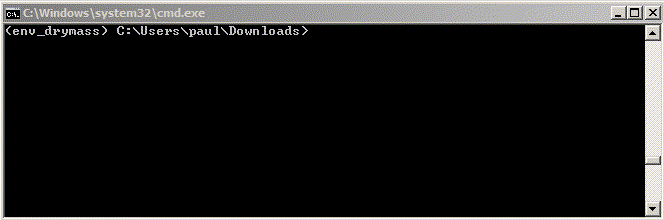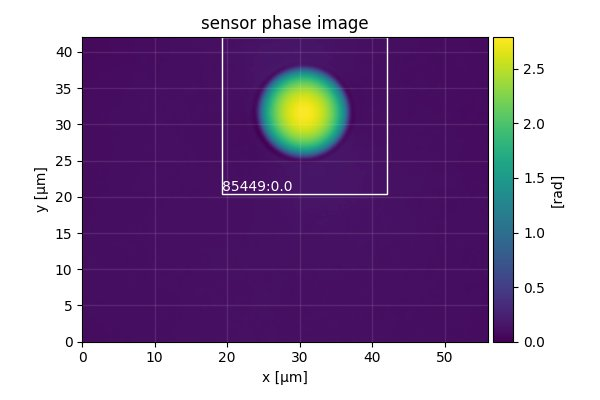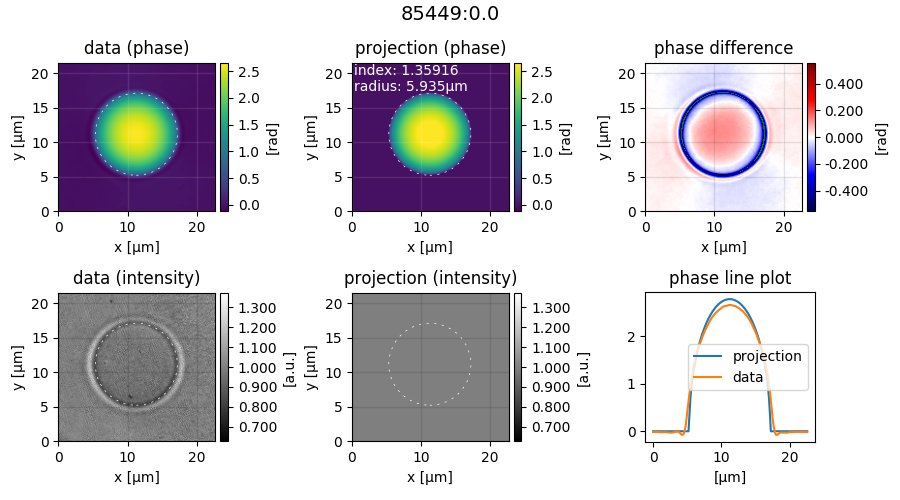## Introduction¶

Microgel beads are transparent, homogeneous, and spherical objects that are ideal test objects for quantitative phase imaging. The DryMass command dm_analyze_sphere can estimate the average refractive index of such homogeneous objects. This is a short tutorial that will reproduce the data presented in supplementary figure 2a of reference [Schuermann2017].

## Prerequisites¶

For this tutorial, you need:

## Execute dm_analyze_sphere¶

DryMass comes with a Command line interface (CLI) which is made available after the installation. We will use the DryMass command dm_analyze_sphere to extract the refractive index values of a population of microgel beads. Using the command shell of your operating system, navigate to the location of QLSR_PAA_beads.zip and execute the command dm_analyze_sphere with QLSR_PAA_beads.zip as an argument. You will be prompted for the refractive index of the surrounding medium (1.335), the detector pixel size in microns (0.14), and the wavelength in nanometers (647). Simply type in these values (press the Enter key to let DryMass acknowledge each input). On Windows, this will look similar to this (2.5x time lapse):DryMass has created a directory called QLSR_PAA_beads.zip_dm (the input argument with _dm appended) which contains the following files

• drymass.cfg: DryMass Configuration file
• roi_data.h5: regions of interest (ROIs)
• roi_data.tif: phase and amplitude data of the ROIs as a tif file
• roi_slices.txt: positions of the ROIs
• sensor_data.h5: full sensor QPI data
• sensor_data.tif: full sensor phase and amplitude data as a tif file
• sensor_roi_images.tif: plotted full sensor phase images with ROIs
• sphere_edge_projection_data.h5: simulated (projection) phase and amplitude data
• sphere_edge_projection_images.tif: visualization of the sphere analysis of the ROIs as a tif file
• sphere_edge_projection_statistics.txt: sphere analysis results as a text file

## Examine the results¶

Let’s have a look at sensor_roi_images.tif (using Fiji or Windows Photo Viewer). This is the first image stored in the tif file:It shows the full sensor phase image of the first bead. The white rectangle indicates the ROI that was found by DryMass, labeled with the identifier 85449:0.0. The file sensor_roi_images.tif allows you to check that DryMass has correctly found the objects that you are interested in. If the beads were not detected correctly, we would probably have to adjust the size parameter in the Configuration file (see also dm_extract_roi).

It appears that DryMass has correctly found all beads with the default settings. Next, open the file sphere_edge_projection_images.tif. The identifier of the first ROI is shown at the top, the first column contains phase and intensity of the experimental data, the second column contains the modeled data (with refractive index and radius used for the simulation), and the third column shows the phase-difference as well as line plots through the phase images.Note that the modeled intensity image is all-one, because the projection model only models the optical thickness and thus only affects the phase data. Also, note that the phase-difference image between data and model only has small deviations in the background phase. If there were large ramp-like structures or offsets, we would have to modify the background correction.

## Post-processing¶

A closer examination of the phase-difference images shows that there seem to be either deformed beads or imaging artifacts in the images with the identifiers (prepend 85449:): 3.0, 6.0, 23.0, 25.0, 26.0, 34.0, 35.0, 38.0, 39.0, 50.0, 51.0, 54.0, 57.0, 59.0, 63.0, 66.0, and 70.0. Due to their asymmetry we ignore these images in our analysis by removing the respective rows from sphere_edge_projection_statistics.txt (Note that this file will be overridden when dm_analyze_sphere is executed again). We can then load the statistics file into a statistical analysis application and compute the average and the standard deviation of the refractive index. In Python, this can be done with

import numpy as np